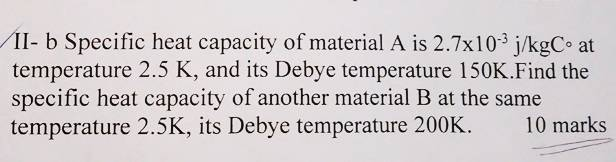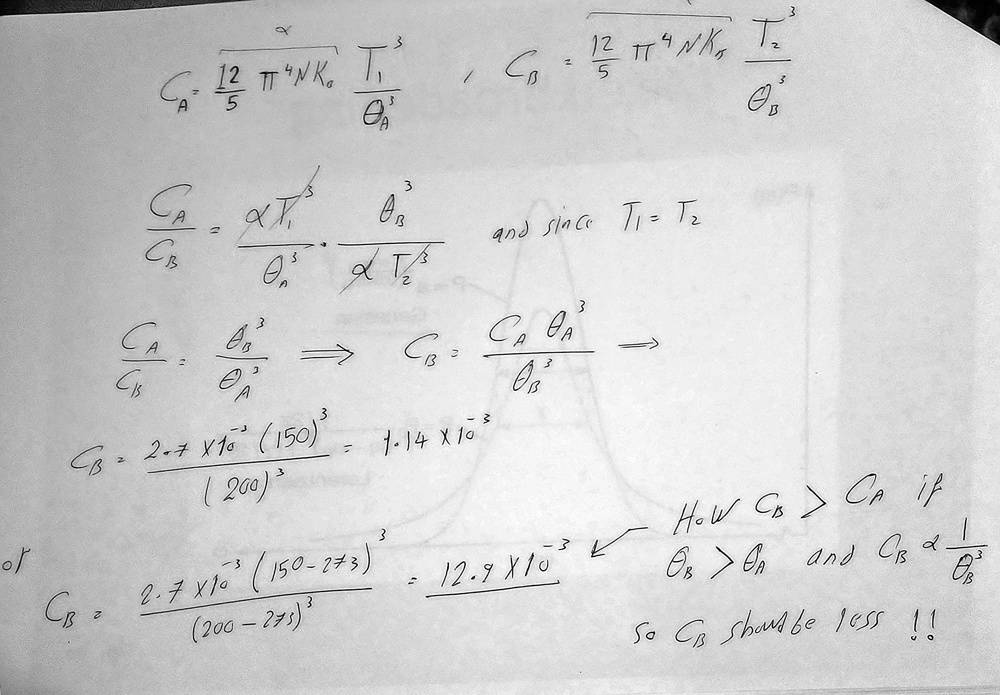# A question about the specific heat and Debye temperature

patric44
Homework Statement:
it was a problem written in a book , asking to calculate the specific heat of a substance given the specific heat of another one and their Debye temperatures
Relevant Equations:
C = constant/Θ^3this is my attempt of a solution , but my only equation is should i convert Θ to Celsius , and if i did the specific heat of the other
substance is greater , how is that if its inversely proportional with temperature ! . and the other Θ is 200 K so it should be less ?!

Gold Member
The heat capacity is defined as the amount of heat to be supplied to a given mass of a material to produce a unit change in its temperature. The unit change in temperature (whether given in ##K## or ##°C##) is the same as they differ only by some constant (here ##273.15 K##).

patric44
The heat capacity is defined as the amount of heat to be supplied to a given mass of a material to produce a unit change in its temperature. The unit change in temperature (whether given in ##K## or ##°C##) is the same as they differ only by some constant (here ##273.15 K##).
so which one is correct the first solution or the second , the fact is that constant is not a multiplying factor ,rather subtraction (T-273) suggest that it will not cancel between the numerator and denominator .
so the ration is not the same .
which one is correct ? and if it was the second one ,why? since CB is larger ! although its inversely proportional with Debye temperature .

Homework Helper
You should not convert Θ to Celsius. Your equation assumes absolute temperatures. You are correct that having a different zero on the temperature scale means the ratio will come out differently.

patric44
You should not convert Θ to Celsius, and if you do, you don't do it by subtracting 273!
so my first answer was correct .
how should i convert it ?

Homework Helper
Sorry, made a mistake there - I've corrected it. Why do you want to convert your answer? To what?
Do you mean you want to convert J/kgK to J/kg°C? How do you think you would do that?
Do you appreciate the difference between converting a temperature value in K to °C (or vice versa), and converting a temperature interval in K to °C?

patric44
Sorry, made a mistake there - I've corrected it. Why do you want to convert your answer? To what?
Do you mean you want to convert J/kgK to J/kg°C? How do you think you would do that?
Do you appreciate the difference between converting a temperature value in K to °C (or vice versa), and converting a temperature interval in K to °C?
i want to convert it because the fact the the specific heat was given with J/kg°C seemed a little tricky after seeing Θ in K , i thought there must be a trick or something . and since the conversion factor is not a multiplication suggests that i should or the ratio will be different.
Isn't the conversion between k to c is just T-273. I don't understand your last question

Homework Helper
The °C and K temperature scales have the same scale factor but a different zero. As T(K) = T(°C) + 273, if you increase T from 283 to 293 K, you increase it from 10°C to 20°C. The increase is 10 degrees in each case, because the scale factor is the same. If you are converting a temperature value (a point on the scale) from K to °C, you subtract 273. If you are converting a temperature difference in K to one in °C, the number is the same.

When you are considering heat capacity, you are considering the heat needed to change the temperature by a given amount. This temperature difference is the same in K or °C, so the heat capacity has the same value in J/kgK or J/kg°C. However, that does not mean that you can use temperature values in K or °C as you choose in the equation. The equation requires absolute temperatures, i.e. in a scale where the zero of the scale comes at absolute zero.

To give another example, T(°F) = 1.8*T(°C) + 32. Here there is both a zero offset and a different scale factor (1.8). This means a temperature difference of 10°C will be the same as a difference of 18°F. You often see in the news statements by reporters who know, e.g., that 10°C = 50°F, and will talk of a temperature of "-10°C (-50°F)" or "the temperature increased by 10°C (50°F)". Can you see why these are wrong?

patric44
Oh you mean that the specific heat is just concerned with the change in temperature which is T2-T1 and the 273 will cancel so its the same as the difference between Celsius.
am I correct